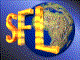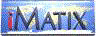| iMatix home page | << | < | > | >>SFLVersion 2.11

### sym_exec_all

```#include "sflsymb.h"
int
sym_exec_all (
const SYMTAB *table,                /*  Symbol table to process          */
symfunc the_function, ...           /*  Function to call                 */
)
```

#### Synopsis

Traverses the symbol table, executing the specified function for every symbol in the table. The function receives one or more arguments: the first argument is a SYMBOL pointer to the symbol, and following arguments as supplied by the caller. Continues so long as the function returns TRUE; halts when every symbol has been processed, or when the function returns FALSE. Returns the number of symbols processed, if all symbols were processed; or negative the number of symbols processed if processing stopped early due to the function returning FALSE or other errors. The symbols are processed in reverse creation order; the newest symbol is processed first.

#### Examples

```    static Bool
dump_symbol (SYMBOL *symbol, ...)
{
printf ("%s = %s\n", symbol-> name, symbol-> value);
return (TRUE);
}

SYMTAB
*table;

table = sym_create_table ();
sym_create_symbol (table, "Symbol 1", "value 1");
sym_create_symbol (table, "Symbol 2", "value 2");
sym_exec_all (table, dump_symbol);
sym_delete_table (table);
```

#### Source Code - (sflsymb.c)

```{
SYMBOL
*symbol;                        /*  Next symbol in table             */
va_list
argptr;                         /*  Argument list pointer            */
int
count = 0;                      /*  Number of symbols processed ok   */
Bool
alldone = TRUE;                 /*  Assume all symbols will be done  */

ASSERT (table);

va_start (argptr, the_function);    /*  Start variable args processing   */
for (symbol = table-> symbols; symbol; symbol = symbol-> next)
{
if ((*the_function) (symbol, argptr))
count++;
else
{
alldone = FALSE;
break;
}
}
va_end (argptr);                    /*  End variable args processing     */

if (alldone)
return (count);                 /*  All symbols processed -> positive*/
else
return (-(count));              /*  Stopped early -> negative count  */
}
```

 | << | < | > | >>Copyright © 1996-2000 iMatix Corporation